# High School Algebra: Properties of Functions Chapter Exam

Exam Instructions:

Choose your answers to the questions and click 'Next' to see the next set of questions. You can skip questions if you would like and come back to them later with the yellow "Go To First Skipped Question" button. When you have completed the practice exam, a green submit button will appear. Click it to see your results. Good luck!

### Page 1

#### Question 4 4. Using the graph, find f(f(-2)).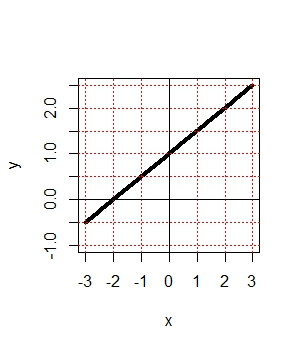#### Question 5 5. Use the graph to find f(f(2)).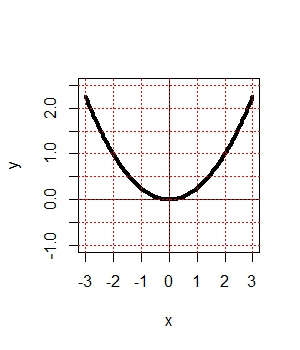### Page 2

#### Question 7 7. Which kind of transformation would change the purple function below into the yellow one?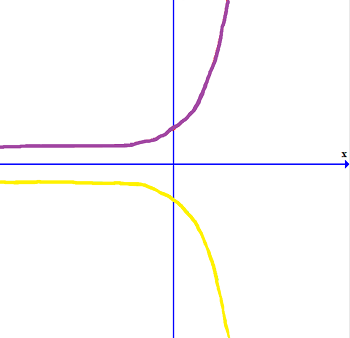### Page 3

#### Question 15 15. In the figure shown below, the graph of the yellow function is a translation of the graph of the blue function. If the expression for the blue function is y(x) = b(x), which of the following is the equation of the yellow function?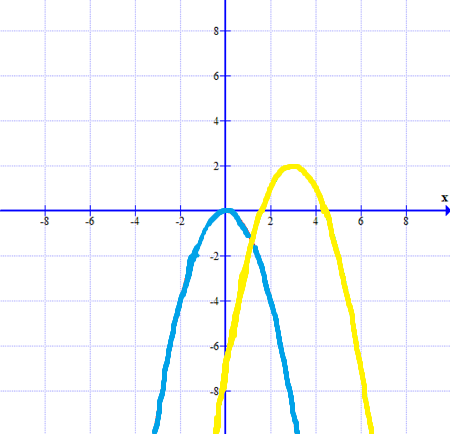### Page 4

#### Question 16 16. What is the domain of the function below?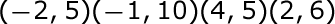#### Question 19 19. What is the inverse function of f(x) = 3(x - 2)3?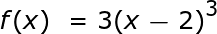#### Question 20 20. The revenue of a company is represented by R(x) and their costs are represented by C(x). What is the profit function R(x) - C(x)?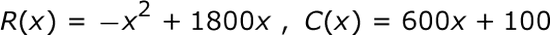### Page 5

#### Question 22 22. Find the domain of f(x)/g(x), if: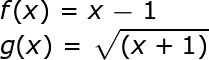#### Question 23 23. What is the range of the function represented by the graph below?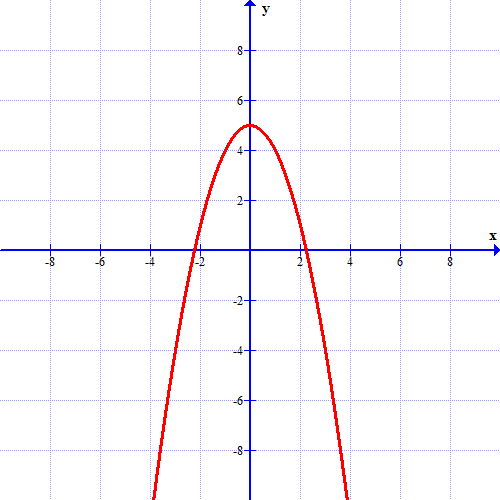### Page 6

#### High School Algebra: Properties of Functions Chapter Exam Instructions

Choose your answers to the questions and click 'Next' to see the next set of questions. You can skip questions if you would like and come back to them later with the yellow "Go To First Skipped Question" button. When you have completed the practice exam, a green submit button will appear. Click it to see your results. Good luck!

Support